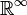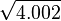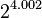# 1617-257/TUT-R-4

On 10/6/16, we showed that$\mathbb R^\infty$ is a metric space where compactness is not equivalent to being closed and bounded. We also showed that$\mathbb R^\infty$ is not complete with respect to the norm we gave it.
We also approximated the values of$\sqrt{4.002}$ and$2^{4.002}$ with first order derivatives.